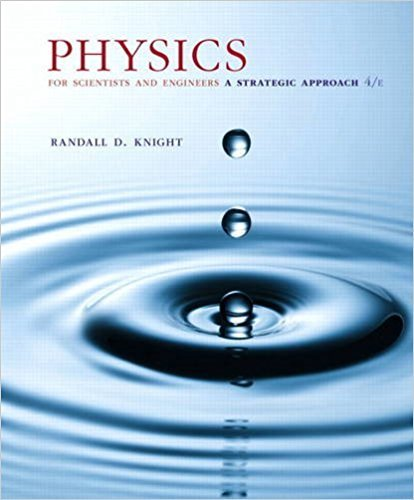×

# FIGURE EX17.28 shows the circular wave fronts emitted byISBN: 9780134081496 191

## Solution for problem 17.62 Chapter 17

Physics for Scientists and Engineers: A Strategic Approach, Standard Edition (Chs 1-36) | 4th Edition

• Textbook Solutions
• 2901 Step-by-step solutions solved by professors and subject experts
• Get 24/7 help from StudySoup virtual teaching assistantsPhysics for Scientists and Engineers: A Strategic Approach, Standard Edition (Chs 1-36) | 4th Edition

4 5 0 394 Reviews
17
0
Problem 17.62

FIGURE EX17.28 shows the circular wave fronts emitted by two wave sources. a. Are these sources in phase or out of phase? Explain. b. Make a table with rows labeled P, Q, and R and columns labeled r1, r2, r, and C/D. Fill in the table for points P, Q, and R, giving the distances as multiples of l and indicating, with a C or a D, whether the interference at that point is constructive or destructive.

Step-by-Step Solution:
Step 1 of 3

Chapter 2: Simple Harmonic Motion 2.1 The Simplest Vibration 1. What is the simplest possible system that demonstrates vibration a. A mass suspended on a spring demonstrates our system at rest: the mass is at the equilibrium position, and...

Step 2 of 3

Step 3 of 3

##### ISBN: 9780134081496

The answer to “FIGURE EX17.28 shows the circular wave fronts emitted by two wave sources. a. Are these sources in phase or out of phase? Explain. b. Make a table with rows labeled P, Q, and R and columns labeled r1, r2, r, and C/D. Fill in the table for points P, Q, and R, giving the distances as multiples of l and indicating, with a C or a D, whether the interference at that point is constructive or destructive.” is broken down into a number of easy to follow steps, and 77 words. Since the solution to 17.62 from 17 chapter was answered, more than 237 students have viewed the full step-by-step answer. The full step-by-step solution to problem: 17.62 from chapter: 17 was answered by , our top Physics solution expert on 12/28/17, 08:06PM. Physics for Scientists and Engineers: A Strategic Approach, Standard Edition (Chs 1-36) was written by and is associated to the ISBN: 9780134081496. This full solution covers the following key subjects: . This expansive textbook survival guide covers 42 chapters, and 4463 solutions. This textbook survival guide was created for the textbook: Physics for Scientists and Engineers: A Strategic Approach, Standard Edition (Chs 1-36), edition: 4.

Unlock Textbook Solution

FIGURE EX17.28 shows the circular wave fronts emitted by

×